Author: Oscar Cronquist Article last updated on January 18, 2023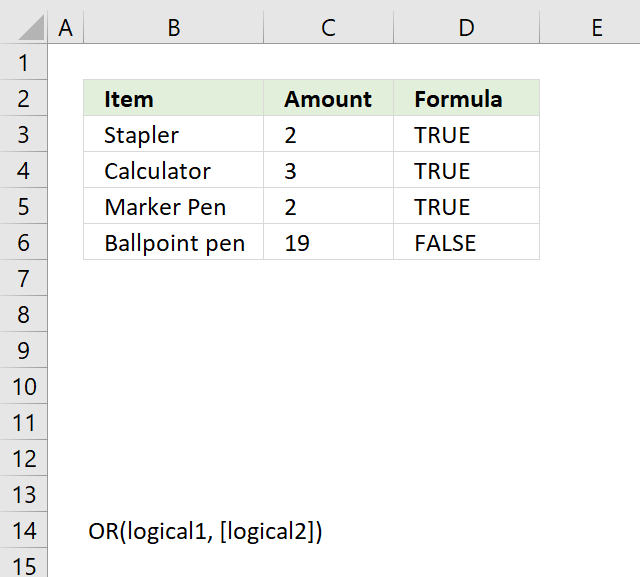The OR function allows you to carry out a logical test in each argument and if at least one argument returns TRUE the OR function returns TRUE.

If all arguments return FALSE the OR function also returns FALSE.

Formula in cell D3:

=OR(B3="Calculator",C3<10)

The picture above shows the formula in column D, it returns TRUE in cell D3, D4 and D5 because the amount is below 10.

## 1. OR function syntax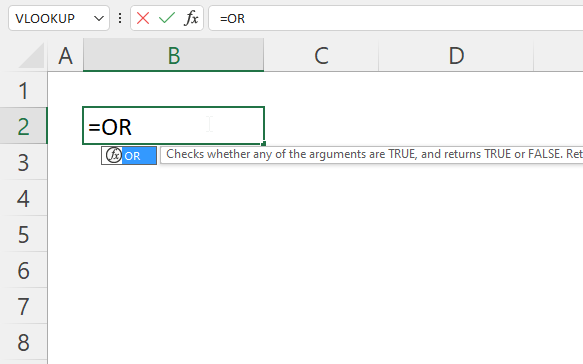OR(logical1, [logical2])

The or function is a logical function, it allows you to evaluate multiple conditions.

It can be used instead of nested IF functions. The OR function is even more versatile if you combine it with the AND function, there is an example below using both the OR and AND functions.

## 2. OR function arguments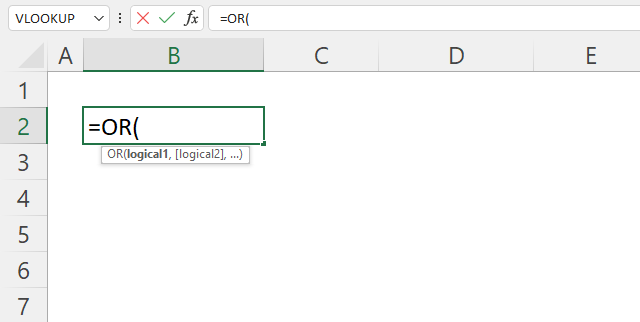logical1 Required. A logical expression that returns a logical value or a number. [logical2] Optional. You can have up to 254 additional arguments.

## 3. Boolean arguments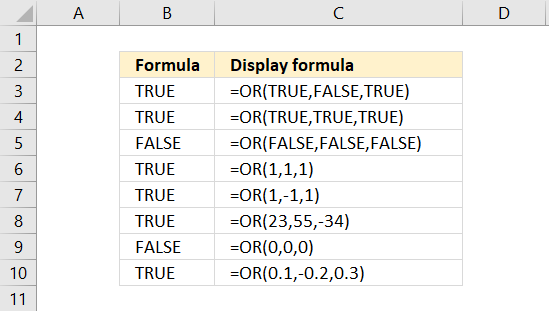The OR function arguments can result in TRUE or FALSE, however, it also treats all numbers, both positive and negative, as TRUE.

The exception to that is 0 (zero) which is treated the same as FALSE.

Formula in cell B3:

=OR(TRUE,FALSE,TRUE)

Formula in cell B3 returns TRUE. TRUE exists at least once.

Formula in cell B4:

=OR(TRUE,TRUE,TRUE)

Formula in cell B4 returns TRUE.

Formula in cell B5:

=OR(FALSE,FALSE,FALSE)

Formula in cell B4 returns FALSE. All arguments are FALSe, OR function returns FALSE.

Formula in cell B6:

=OR(1,1,1)

The numerical equivalent to TRUE is any number other than 0 (zero). 1 is therefore  TRUE. Formula in cell B6 returns TRUE.

Formula in cell C3:

=OR(1,-1,1)

The same goes for negative values. -1 is TRUE.

Formula in cell C3:

=OR(23,55,-34)

23, 55 and -34 are all TRUE.

Formula in cell C3:

=OR(0,0,0)

0 (zero) is FALSE. All arguments are FALSE, OR function returns FALSE.

Formula in cell C3:

=OR(0.1,-0.2,0.3)

All numbers are not zero, they all evaluate to TRUE.

## 4. OR function - multiple conditions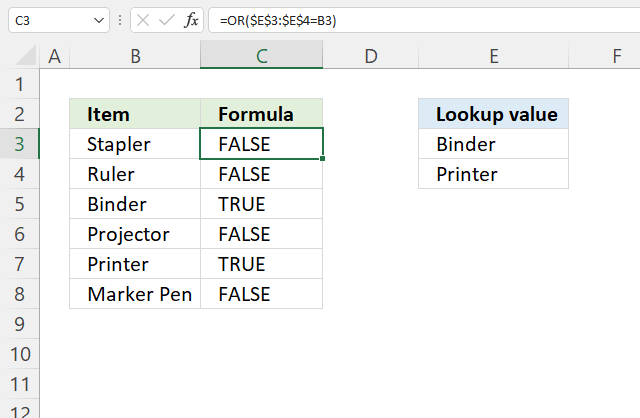The array formula in cell C3 performs two logical tests. If the value in cell B3 is equal to cell E3 or E4 the formula returns TRUE.

Formula in cell C3:

=OR(\$E\$3:\$E\$4=B3)

The technique used in column C that compares a value to multiple values using a cell range instead of declaring each logical expression in an argument saves you time and effort. The downside is that you need to enter the formula as an array formula unless you use Excel 365.

To enter the formula above as an array formula, type the formula in a cell. Press and hold CTRL + SHIFT keys simultaneously, then press Enter once. Release all keys.

The formula is now enclosed with curly brackets, they indicate you successfully entered the formula as an array formula. Don't enter the curly brackets yourself.

### 4.1 Explaining formula in cell C3

#### Step 1 - Reference conditions

You will be using the same conditions in cell C3 and cells below, it is a good idea to create absolute references meaning they won't change when you copy the cell and paste to cells below.

The dollar sign \$ lets you lock both column and row references, see reference below.

\$E\$3:\$E\$4

returns {"Binder";"Printer"}. The curly brackets tell you that this is an array of values, they can have a comma or semicolon delimiting character or both.

#### Step 2 - Compare conditions with cell value

The equal sign is a logical operator that lets you compare value to value or in this case value to values. It returns a boolean value TRUE or FALSE.

\$E\$3:\$E\$4=B3

becomes

{"Binder";"Printer"}="Stapler"

and returns {FALSE; FALSE}. None of the conditions match the value in cell B3.

#### Step 3 - Evaluate OR function

The OR function returns TRUE if at least one of the boolean values in the array is TRUE.

OR(\$E\$3:\$E\$4=B3)

becomes

OR({FALSE; FALSE})

and returns FALSE.

## 5. OR function not working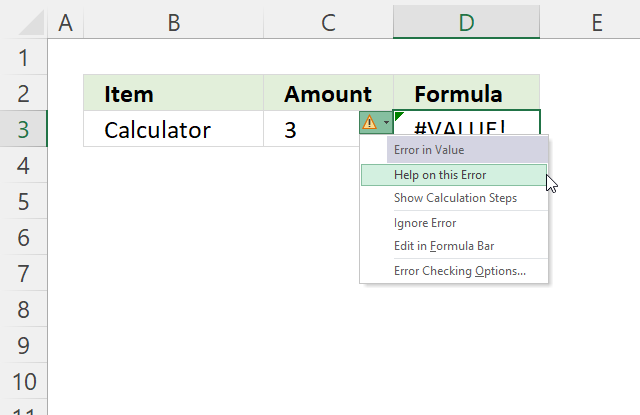The OR function returns an error value if it's not working properly. Press with the mouse on the exclamation mark and a pop-up menu appears.

Press with the mouse on "Help on this error". It will take you to the Microsoft website where you can troubleshoot the formula further.

### 5.1 #NAME! errorThe name error occurs if you misspelled a function. Check the formula in the formula bar. In the example above the OR function is wrong.

### 5.2 Calculation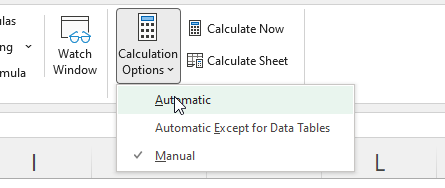Check that the calculation options are set to automatic.

Go to top formulas. On the ribbon. Press with left mouse button on the calculation options button. A pop-up menu appears, select "Automatic".

### 5.3 #VALUE! error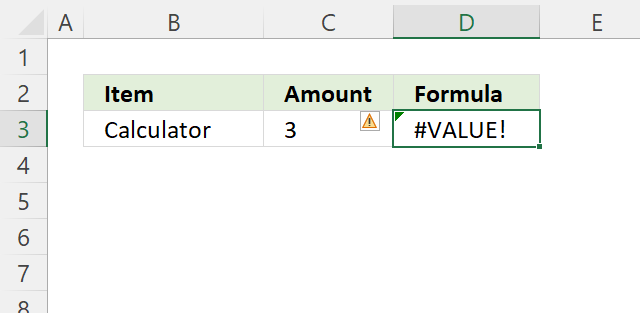Formula in cell D3:

=OR("Calculator", "Pen")

The or function returns a value error if one of the arguments is not a Boolean value or numerical value.

## 6. OR function array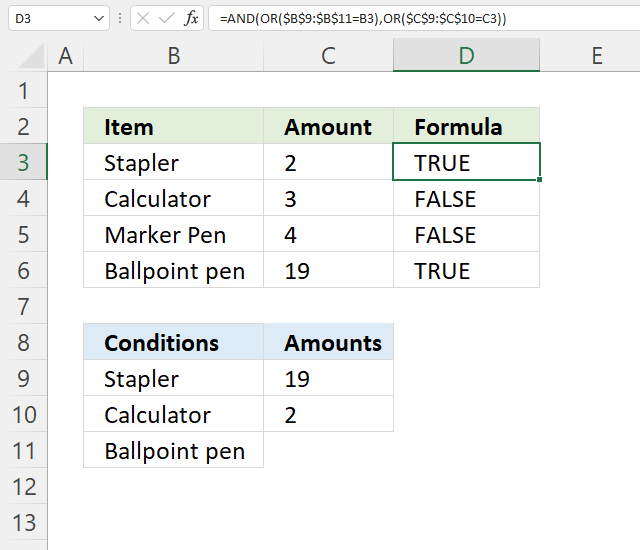The array formula in cell D3 evaluates two logical expressions. If the value in cell B3 is equal to any of the conditions in cells B9:B11 and the value in cell C2 is equal to any of the numbers in cells C9:C10 the formula returns TRUE.

Formula in cell D3:

=AND(OR(\$B\$9:\$B\$11=B3),OR(\$C\$9:\$C\$10=C3))

Cell B3 is equal to a condition in cell B9 and cell C3 is equal to C10, the formula returns TRUE in cell D3.

### 6.1 Explaining formula in cell C3

#### Step 1 - Compare values

The equal sign is a logical operator that lets you compare value to value or in this case value to values. It returns a boolean value TRUE or FALSE.

\$B\$9:\$B\$11=B3

becomes

{"Stapler";"Calculator";"Ballpoint pen"}="Stapler"

and returns

{TRUE; FALSE; FALSE}.

#### Step 2 - Check if any of the conditions match cell B3

The OR function returns TRUE if at least one of the boolean values in the array is TRUE.

OR(\$B\$9:\$B\$11=B3)

becomes

OR({TRUE; FALSE; FALSE})

and returns TRUE.

#### Step 3 - Check next cell C3

OR(\$C\$9:\$C\$10=C3)

becomes

OR({19;2}=2)

becomes

OR({FALSE; TRUE})

and returns TRUE.

#### Step 4 - Check that all conditions evaluate to TRUE

The AND function returns TRUE if all arguments evaluate to TRUE.

AND(OR(\$B\$9:\$B\$11=B3),OR(\$C\$9:\$C\$10=C3))

becomes

AND(TRUE, TRUE)

and returns TRUE.

## 7. OR function COUNTIF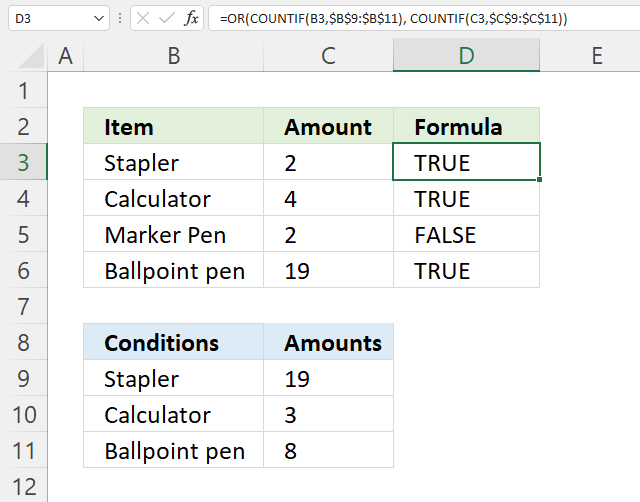The array formula in cell D3 evaluates two logical expressions. If the value in cell B3 is equal to any of the conditions in cells B9:B11 or the value in cell C3 is equal to any of the numbers in cells C9:C11 the formula returns TRUE.

Formula in cell D3:

=OR(COUNTIF(B3,\$B\$9:\$B\$11), COUNTIF(C3,\$C\$9:\$C\$11))

Cell B3 is equal to a condition in cell B9 and cell C3 is not equal to any of the conditions in cells C9:C11, the formula returns TRUE in cell D3.

### 7.1 Explaining formula in cell C3

#### Step 1 - Count values based on first conditions

The COUNTIF function counts the number of cells that meet the condition.

COUNTIF(rangecriteria)

COUNTIF(B3,\$B\$9:\$B\$11)

becomes

COUNTIF("Stapler", {"Stapler"; "Calculator"; "Ballpoint pen"})

and returns {1; 0; 0}.

#### Step 2 - Count values based on second conditions

COUNTIF(C3,\$C\$9:\$C\$11)

becomes

COUNTIF(2, {19; 3; 8})

and returns {0; 0; 0}.

#### Step 3 - Count values

The OR function returns TRUE if at least one value is TRUE.

OR(COUNTIF(B3,\$B\$9:\$B\$11), COUNTIF(C3,\$C\$9:\$C\$11))

becomes

OR({1; 0; 0}, {0; 0; 0})

and returns TRUE. 1 is the numerical equivalent to TRUE.

## 8. OR function with IF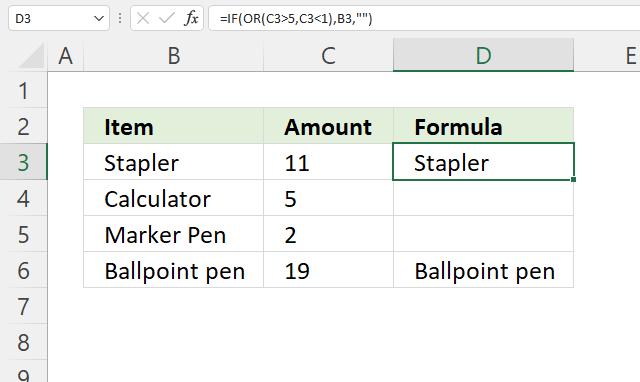Formula in cell D3:

=IF(OR(C3>5,C3<1),B3,"")

### 8.1 Explaining formula in cell C3

#### Step 1 - Check the first condition

The larger than character is a logical operator, it checks if a value is larger than another value. It returns boolean value TRUE or FALSE.

C3>5

becomes

11>5

and returns TRUE.

#### Step 2 - Check the second condition

The smaller than character is a logical operator, it checks if a value is smaller than another value. It returns boolean value TRUE or FALSE.

C3<1

becomes

11<1

and returns FALSE.

#### Step 3 - Evaluate OR function

OR(C3>5,C3<1)

becomes

OR(TRUE, FALSE)

and returns TRUE.

#### Step 4 - Evaluate IF function

The IF function returns one value if the logical test is TRUE and another value if the logical test is FALSE.

IF(logical_test, [value_if_true], [value_if_false])

IF(OR(C3>5,C3<1),B3,"")

## 9. OR function contains text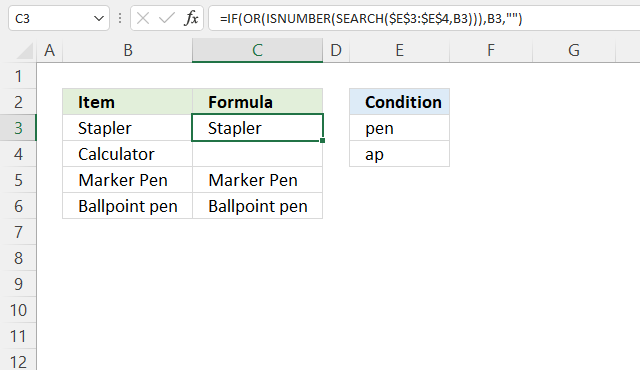Formula in cell D3:

=IF(OR(ISNUMBER(SEARCH(\$E\$3:\$E\$4,B3))),B3,"")

### 9.1 Explaining formula in cell C3

#### Step 1 - Search value for strings

The SEARCH function returns the position of the character at which a specific text string is found. Luckily the IF function accepts any number as TRUE except 0 (zero), however, the SEARCH function returns an error #VALUE! if it can't find the text string.

SEARCH(\$E\$3:\$E\$4,B3)

becomes

SEARCH({"pen";"ap"},"Stapler")

and returns {#VALUE!; 3}.

#### Step 2 - Check if number

To avoid the error value I use the ISNUMBER function that returns TRUE if a number and FALSE if anything else, also formula errors.

ISNUMBER(SEARCH(\$E\$3:\$E\$4,B3))

becomes

ISNUMBER({#VALUE!; 3})

and returns {FALSE; TRUE}

#### Step 3 - Evaluate OR function

OR(ISNUMBER(SEARCH(\$E\$3:\$E\$4,B3)))

becomes

OR({FALSE; TRUE})

and returns TRUE.

#### Step 3 - Evaluate IF function

The IF function returns one value if the logical test is TRUE and another value if the logical test is FALSE.

IF(logical_test, [value_if_true], [value_if_false])

IF(OR(ISNUMBER(SEARCH(\$E\$3:\$E\$4,B3))),B3,"")

becomes

IF(TRUE,B3,"")

and returns "Stapler".

## 10. OR function Conditional Formatting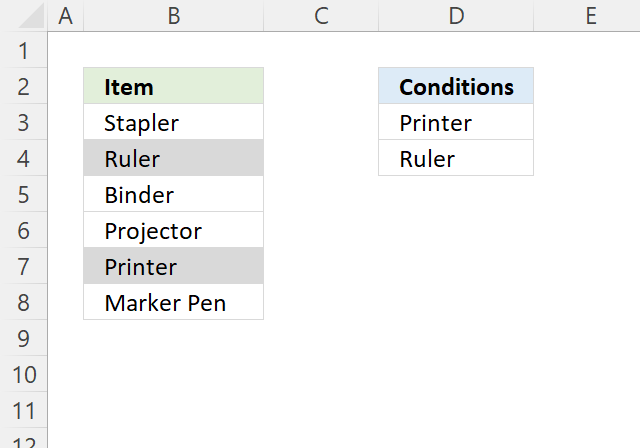The image above shows Conditional Formatting highlgihting cells in ceolumn B if they match the conditions specified in column D.

Conditional formatting formula applied to cell range B3:B8:

=OR(B3=\$D\$3:\$D\$4)

### 10.1 Explaining conditional formatting formula

#### Step 1 - Define reference to conditions

This reference must stay unchanged or locked to D3:D4, use the dollar sign character to lock a reference for both column and row.

\$D\$3:\$D\$4

#### Step 2 - Compare values

The equal sign is a logical operator that lets you compare value to value or in this case value to values. It returns a boolean value TRUE or FALSE.

B3=\$D\$3:\$D\$4

becomes

"Stapler"={"Printer"; "Ruler"}

and returns {FALSE; FALSE}

#### Step 3 - Evaluate logical tests

OR(B3=\$D\$3:\$D\$4)

becomes

OR({FALSE; FALSE})

and returns FALSE.

### 10.2 How to apply Conditional formatting

How to apply Conditional Formatting